#Euler's FormulaThe beautiful and perhaps mysterious formula of Euler which is the subject of this section is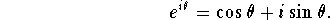Several questions might immediately come to mind.

What does an exponential function have to do with trigonometric functions? At the pre-calculus level we are familiar with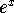as a function which increases rapidly as x grows, and with the oscillatory nature of the trigonometric functions.

How do we make sense of raising a real number to an imaginary power? Our rules of arithmetic have only told us how to extend addition and multiplication from the real numbers to the complex numbers.

We will eventually give a complete and airtight answer to these questions in the section on complex functions(link), but we can get acquainted with Euler's formula and strip away some of its mystery by extrapolating a few simple properties of the real function.

First, if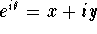then the equation which we obtain by replacing i with -i should also be true. After all -i is as good a square root of -1 as i.

So we should also have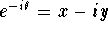. And if we multiply these together,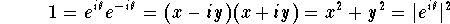so that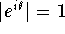So we know at least that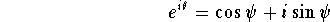for some angle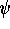. But does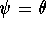?

Recall that the exponential functionis well approximated by the linear function 1+x when x is very small. (Try it on a calculator.) We will now assume that the function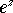retains this property for complex z with small modulus.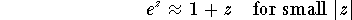so in particular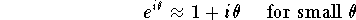And now we use two more approximations which are very good for small values of. (try it)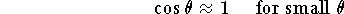and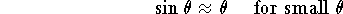These approximations become increasingly good as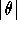decreases and it is clear that Euler's formula holds when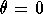.

Putting all this together we boldly claim: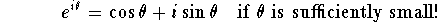Notice that by de Moivre's formula from the previous section, this means that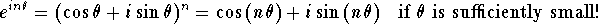And since any angle can be written as an integer multiplied by something ``sufficiently small!'', our claim is bold indeed.

What we should notice at this stage, is that if we want to extend the functionto complex values of x in a way consistent with what we already know about the function, then it is very reasonable to expect oscillatory behavior from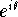and perhaps also reasonable to accept Euler's lovely formula.

We will soon analyze and understand the formula completely.[Algebra] [Complex Variables]
[Geometry] [Trigonometry ]
[Calculus] [Differential Equations] [Matrix Algebra]S.O.S MATHematics home page

Do you need more help? Please post your question on our S.O.S. Mathematics CyberBoard.Author: Michael O'Neill